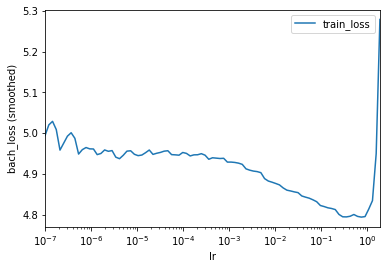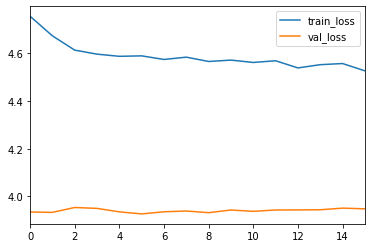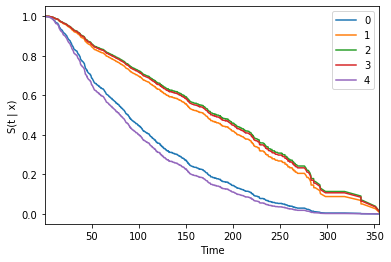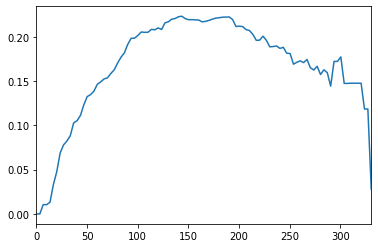# Cox-PH and DeepSurv¶

In this notebook we will train the Cox-PH method, also known as DeepSurv. We will use the METABRIC data sets as an example

A more detailed introduction to the pycox package can be found in this notebook about the LogisticHazard method.

The main benefit Cox-CC (and the other Cox methods) has over Logistic-Hazard is that it is a continuous-time method, meaning we do not need to discretize the time scale.

In :
import numpy as np
import matplotlib.pyplot as plt
from sklearn.preprocessing import StandardScaler
from sklearn_pandas import DataFrameMapper

import torch
import torchtuples as tt

from pycox.datasets import metabric
from pycox.models import CoxPH
from pycox.evaluation import EvalSurv

In :
## Uncomment to install sklearn-pandas
# ! pip install sklearn-pandas

In :
np.random.seed(1234)
_ = torch.manual_seed(123)


## Dataset¶

We load the METABRIC data set and split in train, test and validation.

In :
df_train = metabric.read_df()
df_test = df_train.sample(frac=0.2)
df_train = df_train.drop(df_test.index)
df_val = df_train.sample(frac=0.2)
df_train = df_train.drop(df_val.index)

In :
df_train.head()

Out:
x0 x1 x2 x3 x4 x5 x6 x7 x8 duration event
0 5.603834 7.811392 10.797988 5.967607 1.0 1.0 0.0 1.0 56.840000 99.333336 0
1 5.284882 9.581043 10.204620 5.664970 1.0 0.0 0.0 1.0 85.940002 95.733330 1
3 6.654017 5.341846 8.646379 5.655888 0.0 0.0 0.0 0.0 66.910004 239.300003 0
4 5.456747 5.339741 10.555724 6.008429 1.0 0.0 0.0 1.0 67.849998 56.933334 1
5 5.425826 6.331182 10.455145 5.749053 1.0 1.0 0.0 1.0 70.519997 123.533333 0

## Feature transforms¶

We have 9 covariates, in addition to the durations and event indicators.

We will standardize the 5 numerical covariates, and leave the binary variables as is. As variables needs to be of type 'float32', as this is required by pytorch.

In :
cols_standardize = ['x0', 'x1', 'x2', 'x3', 'x8']
cols_leave = ['x4', 'x5', 'x6', 'x7']

standardize = [([col], StandardScaler()) for col in cols_standardize]
leave = [(col, None) for col in cols_leave]

x_mapper = DataFrameMapper(standardize + leave)

In :
x_train = x_mapper.fit_transform(df_train).astype('float32')
x_val = x_mapper.transform(df_val).astype('float32')
x_test = x_mapper.transform(df_test).astype('float32')


We need no label transforms

In :
get_target = lambda df: (df['duration'].values, df['event'].values)
y_train = get_target(df_train)
y_val = get_target(df_val)
durations_test, events_test = get_target(df_test)
val = x_val, y_val


## Neural net¶

We create a simple MLP with two hidden layers, ReLU activations, batch norm and dropout. Here, we just use the torchtuples.practical.MLPVanilla net to do this.

Note that we set out_features to 1, and that we have not output_bias.

In :
in_features = x_train.shape
num_nodes = [32, 32]
out_features = 1
batch_norm = True
dropout = 0.1
output_bias = False

net = tt.practical.MLPVanilla(in_features, num_nodes, out_features, batch_norm,
dropout, output_bias=output_bias)


## Training the model¶

To train the model we need to define an optimizer. You can choose any torch.optim optimizer, but here we instead use one from tt.optim as it has some added functionality. We use the Adam optimizer, but instead of choosing a learning rate, we will use the scheme proposed by Smith 2017 to find a suitable learning rate with model.lr_finder. See this post for an explanation.

In :
model = CoxPH(net, tt.optim.Adam)

In :
batch_size = 256
lrfinder = model.lr_finder(x_train, y_train, batch_size, tolerance=10)
_ = lrfinder.plot()In :
lrfinder.get_best_lr()

Out:
0.07390722033525823

Often, this learning rate is a little high, so we instead set it manually to 0.01

In :
model.optimizer.set_lr(0.01)


We include the EarlyStopping callback to stop training when the validation loss stops improving. After training, this callback will also load the best performing model in terms of validation loss.

In :
epochs = 512
callbacks = [tt.callbacks.EarlyStopping()]
verbose = True

In :
%%time
log = model.fit(x_train, y_train, batch_size, epochs, callbacks, verbose,
val_data=val, val_batch_size=batch_size)

0:	[0s / 0s],		train_loss: 4.7557,	val_loss: 3.9349
1:	[0s / 0s],		train_loss: 4.6736,	val_loss: 3.9334
2:	[0s / 0s],		train_loss: 4.6137,	val_loss: 3.9538
3:	[0s / 0s],		train_loss: 4.5968,	val_loss: 3.9501
4:	[0s / 0s],		train_loss: 4.5877,	val_loss: 3.9355
5:	[0s / 0s],		train_loss: 4.5896,	val_loss: 3.9269
6:	[0s / 0s],		train_loss: 4.5745,	val_loss: 3.9359
7:	[0s / 0s],		train_loss: 4.5842,	val_loss: 3.9391
8:	[0s / 0s],		train_loss: 4.5660,	val_loss: 3.9321
9:	[0s / 0s],		train_loss: 4.5719,	val_loss: 3.9433
10:	[0s / 0s],		train_loss: 4.5620,	val_loss: 3.9377
11:	[0s / 0s],		train_loss: 4.5691,	val_loss: 3.9434
12:	[0s / 0s],		train_loss: 4.5390,	val_loss: 3.9436
13:	[0s / 0s],		train_loss: 4.5526,	val_loss: 3.9444
14:	[0s / 0s],		train_loss: 4.5575,	val_loss: 3.9511
15:	[0s / 0s],		train_loss: 4.5269,	val_loss: 3.9482
CPU times: user 728 ms, sys: 109 ms, total: 837 ms
Wall time: 651 ms

In :
_ = log.plot()We can get the partial log-likelihood

In :
model.partial_log_likelihood(*val).mean()

Out:
-4.979708194732666

## Prediction¶

For evaluation we first need to obtain survival estimates for the test set. This can be done with model.predict_surv which returns an array of survival estimates, or with model.predict_surv_df which returns the survival estimates as a dataframe.

However, as CoxPH is semi-parametric, we first need to get the non-parametric baseline hazard estimates with compute_baseline_hazards.

Note that for large datasets the sample argument can be used to estimate the baseline hazard on a subset.

In :
_ = model.compute_baseline_hazards()

In :
surv = model.predict_surv_df(x_test)

In :
surv.iloc[:, :5].plot()
plt.ylabel('S(t | x)')
_ = plt.xlabel('Time')## Evaluation¶

We can use the EvalSurv class for evaluation the concordance, brier score and binomial log-likelihood. Setting censor_surv='km' means that we estimate the censoring distribution by Kaplan-Meier on the test set.

In :
ev = EvalSurv(surv, durations_test, events_test, censor_surv='km')

In :
ev.concordance_td()

Out:
0.6542846245126293
In :
time_grid = np.linspace(durations_test.min(), durations_test.max(), 100)
_ = ev.brier_score(time_grid).plot()In :
ev.integrated_brier_score(time_grid)

Out:
0.16736877357426813
In :
ev.integrated_nbll(time_grid)

Out:
0.4951448893250452
In [ ]: